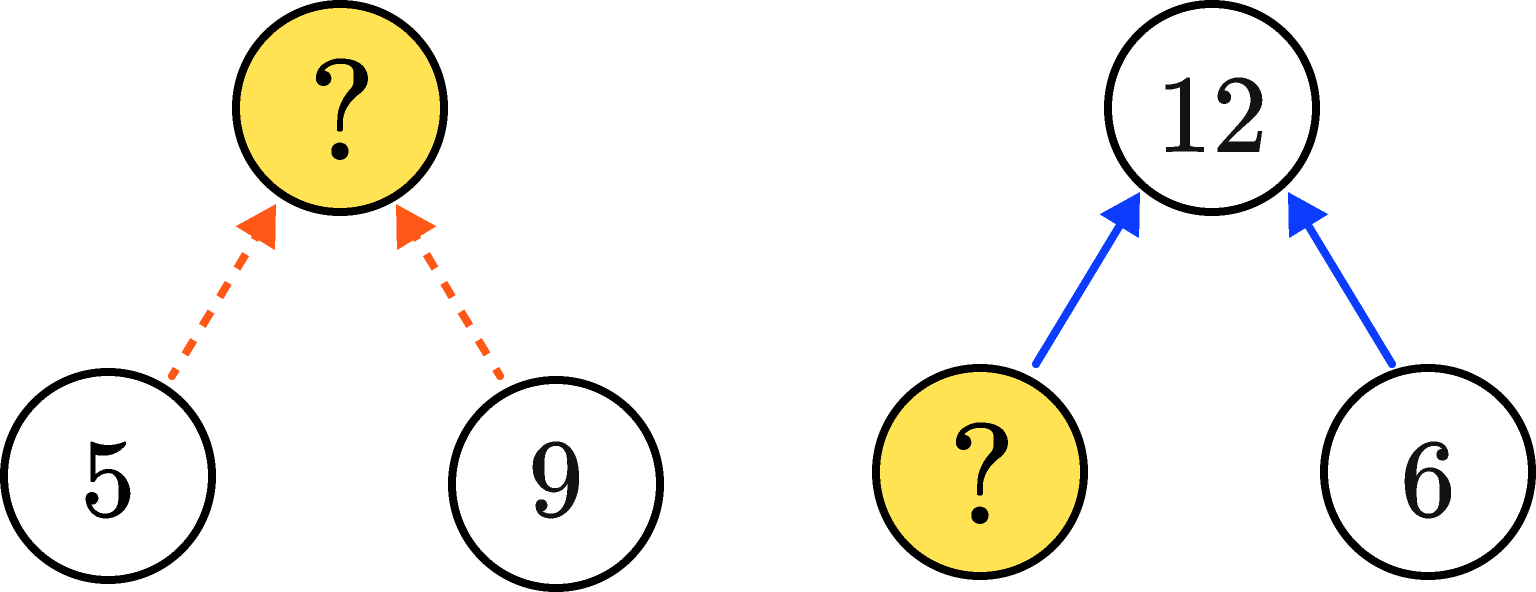## Start in the Middle

In a circle puzzle like the ones below, dashed arrows mean to add and solid arrows mean to multiply. For example, the solution to the puzzle on the left is a number whose sum is $5 + 9,$ which is $14.$ The solution to the puzzle on the right is a number that, when multiplied by $6,$ gives us $12.$ By working backwards, we get a solution of $2.$Keep reading to see a few more complicated example puzzles, or dive right into today's challenge if you feel ready.